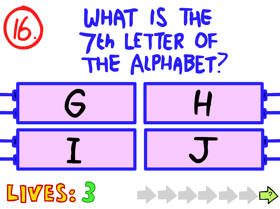## FANDOM

478 Pages

 The Impossible Quiz << Previous Question Question 16 Next Question >>
Question 16Difficulty

Somewhat easy

Reference(s)

None

Question 16 from the Impossible Quiz says "What is the 7th letter of the alphabet?", with the possible choices being "G", "H", "I", and "J".

There's a trick in this question. Though "G" happens to be the seventh letter in alphabetic order, the answer is NOT "G". The actual answer to this question is "H", which is the 7th letter within the words "the alphabet".

## Trivia

• Question 8 from The Impossible Quiz Book reuses this question, albeit with four different options, none of them being "H", but with one of them hinting at the fact that the question does sound familiar. The answer in this case is to press H on your keyboard.
• Question 63 from The Impossible Quiz 2 references this question, and the correct answer from this Question (H) returns in that question, however, you are asked what is the 17th letter of the alphabet rather than the 7th, and there's no trick that would make the H the correct answer